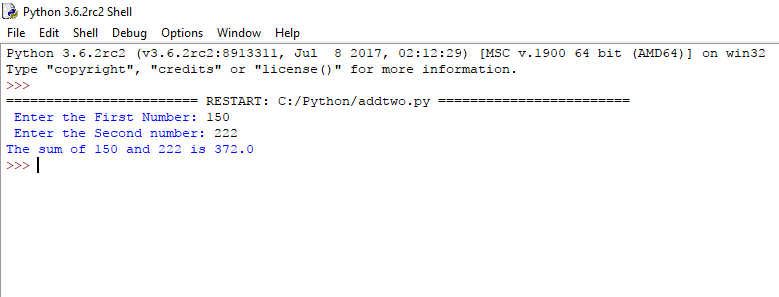# Python Basics : How To Add Numbers

In order to show, we are going to add two numbers using Arithmetic Operators. This simple Python program allows the user to enter two values and then add those two numbers and display the total variable sum.

Python Code :-

```#python program to Add Two Numbers

number1 = input(" Enter the First No: ")

number2 = input(" Enter the Second No: ")

#using arithmetic + Operator to add two numbers

sum = float(number1) + float(number2)

print('The sum of {0} and {1} is {2}'.format(number1, number2, sum))```
Output :-